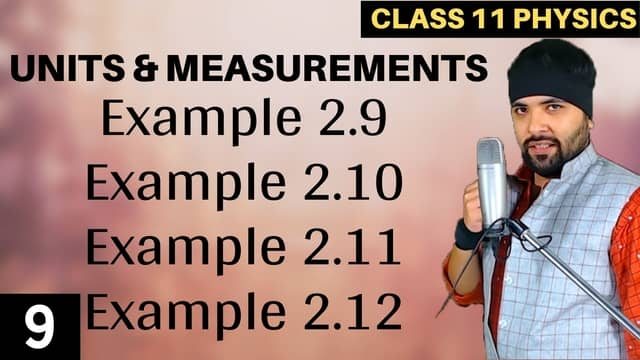# L9 – Example 2.9 to 2.12 Units and Measurements## Topics/Questions discussed in this video:

Example 2.10 Two resistors of resistances R1 = 100±3 ohm and R2 = 200 ± 4 ohm are connected
(a) in series,
(b) in parallel.
Find the equivalent resistance of the
(a) series combination,
(b) parallel combination.
Use for (a) the relation R = R1 + R2 and for (b) \frac{1}{R} = \frac{1}{R_1} + \frac{1}{R_2} and \frac{\Delta R}{R`^{2}} = \frac{\Delta R_1}{{R_1}^2} + \frac{\Delta R_2}{{R_2}^2}

Example 2.9 The resistance R = V/I where V = (100 ± 5)V and I = (10 ± 0.2)A. Find the percentage error in R.

Example 2.11 Find the relative error in Z, if Z = \frac{A^4 B^{\frac{1}{3}}}{C D^{\frac{3}{2}}}.

Example 2.12 The period of oscillation of a simple pendulum is T = 2 \pi \sqrt{\frac{L}{g}}
Measured value of L is 20.0 cm known to 1 mm accuracy and time for 100 oscillations of the pendulum is found to be 90 s using a wrist watch of 1 s resolution. What is the accuracy in the determination of g ?

#### Categories## Agam Sir

Teacher

Ashish Kumar a.k.a Agam Sir has vast experience of 12+ years of teaching Mathematics and Physics. He has skills (academic and vocational) that go with working alongside people, especially young people, their parent(s) and the institutes that works with him. He always seek to be an agent of positive change and progression, a mentor/ trainer/ educator for learners/students, seeing that their needs are ideally met.